## 12.3.5 Probabilistic Localization and Mapping

The problems considered so far in Section 12.3 have avoided probabilistic modeling. Suppose here that probabilistic models exist for the state transitions and the observations. Many problems can be formulated by replacing the nondeterministic models in Section 12.3.1 by probabilistic models. This would lead to probabilistic I-states that represent distributions over a set of possible grids and a configuration within each grid. If the problem is left in its full generality, the I-space is enormous to the point that is seems hopeless to approach problems in the manner used to far. If optimality is not required, then in some special cases progress may be possible.

The current problem is to construct a map of the environment while simultaneously localizing the robot with the respect to the map. Recall Figure 1.7 from Section 1.2. The section covers a general framework that has been popular in mobile robotics in recent years (see the literature suggested at the end of the chapter). The discussion presented here can be considered as a generalization of the discussion from Section 12.2.3, which was only concerned with the localization portion of the current problem. Now the environment is not even known. The current problem can be interpreted as localization in a state space defined as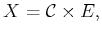(12.29)

in whichis a configuration space and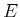is the environment space. A state,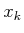, is represented as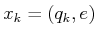; there is nosubscript for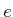because the environment is assumed to be static). The history I-state provides the data to use in the process of determining the state. As for localization in Section 12.2, there are both passive and active versions of the problem. An incremental version of the active problem is sometimes called the next-best-view problem [66,238,793]. The difficulty is that the robot has opposing goals of: 1) trying to turn on the sensor at places that will gain as much new data as possible, and 2) this minimization of redundancy can make it difficult to fuse all of the measurements into a global map. The passive problem will be described here; the methods can be used to provide information for solving the active problem.

Suppose that the robot is a point that translates and rotates in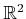. According to Section 4.2, this yields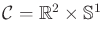, which represents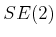. Let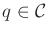denote a configuration, which yields the position and orientation of the robot. Assume that configuration transitions are modeled probabilistically, which requires specifying a probability density,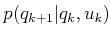. This can be lifted to the state space to obtain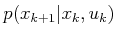by assuming that the configuration transitions are independent of the environment (assuming no collisions ever occur). This replaces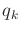and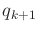byand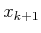, respectively, in whichand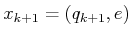for any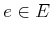.

Suppose that observations are obtained from a depth sensor, which ideally would produce measurements like those shown in Figure 11.15b; however, the data are assumed to be noisy. The probabilistic model discussed in Section 12.2.3 can be used to define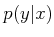. Now imagine that the robot moves to several parts of the environment, such as those shown in Figure 11.15a, and performs a sensor sweep in each place. If the configurationis not known from which each sweep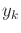was performed, how can the data sets be sewn together to build a correct, global map of the environment? This is trivial after considering the knowledge of the configurations, but without it the problem is like putting together pieces of a jigsaw puzzle. Thus, the important data in each stage form a vector,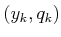. If the sensor observations,, are not tagged with a configuration,, from which they are taken, then the jigsaw problem arises. If information is used to tightly constrain the possibilities for, then it becomes easier to put the pieces together. This intuition leads to the following approach.

Subsections
Steven M LaValle 2020-08-14